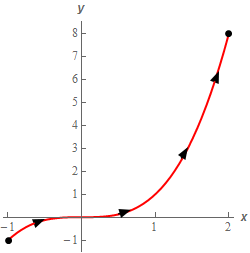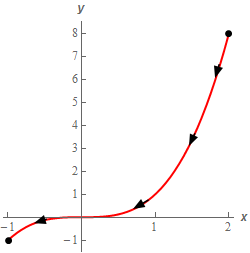Paul's Online Notes
Home / Calculus III / Line Integrals / Line Integrals - Part I
Show Mobile Notice Show All Notes Hide All Notes
Mobile Notice
You appear to be on a device with a "narrow" screen width (i.e. you are probably on a mobile phone). Due to the nature of the mathematics on this site it is best views in landscape mode. If your device is not in landscape mode many of the equations will run off the side of your device (should be able to scroll to see them) and some of the menu items will be cut off due to the narrow screen width.

### Section 16.2 : Line Integrals - Part I

Evaluate $$\displaystyle \int\limits_{C}{{2{x^3}\,ds}}$$ for each of the following curves.

1. $$C$$ is the portion $$y = {x^3}$$ from $$x = - 1$$ to $$x = 2$$.
2. $$C$$ is the portion $$y = {x^3}$$ from $$x = 2$$ to $$x = - 1$$.
Show All Solutions Hide All Solutions

a $$C$$ is the portion $$y = {x^3}$$ from $$x = - 1$$ to $$x = 2$$. Show All Steps Hide All Steps
Start Solution

Let’s start off with a quick sketch of the curve for this part of the problem.For reasons that will become apparent once we get to the second part of this problem let’s call this curve $${C_1}$$ instead of $$C$$. Here then is the parameterization of $${C_1}$$.

$${C_1}:\,\,\vec r\left( t \right) = \left\langle {t,{t^3}} \right\rangle \hspace{0.25in}\,\,\,\,\,\,\, - 1 \le t \le 2$$

Show Step 2

Here is the line integral for this curve.

\begin{align*}\int\limits_{{{C_1}}}{{2{x^3}\,ds}} & = \int_{{ - 1}}^{2}{{2{{\left( t \right)}^3}\sqrt {{{\left( 1 \right)}^2} + {{\left( {3{t^2}} \right)}^2}} \,dt}}\\ & = \int_{{ - 1}}^{2}{{2{t^3}\sqrt {1 + 9{t^4}} \,dt}}\\ & = \left. {\frac{1}{{27}}{{\left( {1 + 9{t^4}} \right)}^{\frac{3}{2}}}} \right|_{ - 1}^2 = \require{bbox} \bbox[2pt,border:1px solid black]{{\frac{1}{{27}}\left( {{{145}^{\frac{3}{2}}} - {{10}^{\frac{3}{2}}}} \right) = 63.4966}}\end{align*}

b $$C$$ is the portion $$y = {x^3}$$ from $$x = 2$$ to $$x = - 1$$. Show All Steps Hide All Steps
Start Solution

Now, as we did in the previous part let’s “rename” this curve as $${C_2}$$ instead of $$C$$.

Next, note that this curve is just the curve from the first step with opposite direction. In other words what we have here is that $${C_2} = - {C_1}$$. Here is a quick sketch of $${C_2}$$ for the sake of completeness.Show Step 2

Now, at this point there are two different methods we could use to evaluate the integral.

The first method is use the fact from the notes that if we switch the directions for a curve then the value of this type of line integral doesn’t change. Using this fact along with the relationship between the curve from this part and the curve from the first part, i.e. $${C_2} = - {C_1}$$ , the line integral is just,

$\int\limits_{{{C_2}}}{{2{x^3}\,ds}} = \int\limits_{{ - {C_1}}}{{2{x^3}\,ds}} = \int\limits_{{{C_1}}}{{2{x^3}\,ds}} = \require{bbox} \bbox[2pt,border:1px solid black]{{\frac{1}{{27}}\left( {{{145}^{\frac{3}{2}}} - {{10}^{\frac{3}{2}}}} \right) = 63.4966}}$

Note that the first equal sign above was just acknowledging the relationship between the two curves. The second equal sign is where we used the fact from the notes.

This is the “easy” method for doing this problem. Alternatively we could parameterize up the curve and compute the line integral directly. We will do that for the rest of this problem just to show how we would go about doing that.

Show Step 3

Now, if we are going to parameterize this curve, and follow the indicated direction, we can’t just use the parameterization from the first part and then “flip” the limits on the integral to “go backwards”. Line integrals just don’t work that way. The limits on the line integrals need to go from smaller value to larger value.

We need a new parameterization for this curve that will follow the curve in the indicated direction. Luckily that is actually pretty simple to do in this case. All we need to do is let $$x = - t$$ as $$t$$ ranges from $$t = - 2$$ to $$t = 1$$. In this way as $$t$$ increases $$x$$ will go from $$x = 2$$ to $$x = - 1$$. In other words, at $$t$$ increases $$x$$ will decrease as we need it to in order to follow the direction of the curve.

Now that we have $$x$$ taken care of the $$y$$ is easy because we know the equation of the curve. To get the parametric equation for $$y$$ all we need to do is plug in the parametric equation for $$x$$ into the equation of the curve. Or,

$y = {\left( { - t} \right)^3} = - {t^3}$

Putting all of this together we get the following parameterization of the curve.

$${C_2}:\,\,\vec r\left( t \right) = \left\langle { - t - {t^3}} \right\rangle \hspace{0.25in}\,\,\,\,\,\,\, - 2 \le t \le 1$$

Show Step 4

Now all we need to do is compute the line integral.

\begin{align*}\int\limits_{{{C_2}}}{{2{x^3}\,ds}} & = \int_{{ - 2}}^{1}{{2{{\left( { - t} \right)}^3}\sqrt {{{\left( { - 1} \right)}^2} + {{\left( { - 3{t^2}} \right)}^2}} \,dt}}\\ & = \int_{{ - 2}}^{1}{{ - 2{t^3}\sqrt {1 + 9{t^4}} \,dt}}\\ & = \left. { - \frac{1}{{27}}{{\left( {1 + 9{t^4}} \right)}^{\frac{3}{2}}}} \right|_{ - 2}^1 = \require{bbox} \bbox[2pt,border:1px solid black]{{ - \frac{1}{{27}}\left( {{{10}^{\frac{3}{2}}} - {{145}^{\frac{3}{2}}}} \right) = 63.4966}}\end{align*}

So, the line integral from this part had exactly the same value as the line integral from the first part as we expected it to.